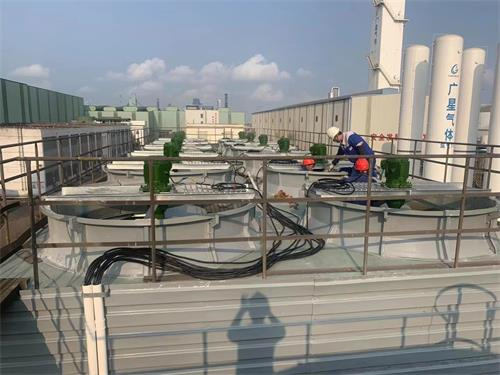﻿ 冷却塔型号及参数有哪些,100T逆流方型冷却塔主要参数_广东特菱空调

# 冷却塔型号及参数有哪些,100T逆流方型冷却塔主要参数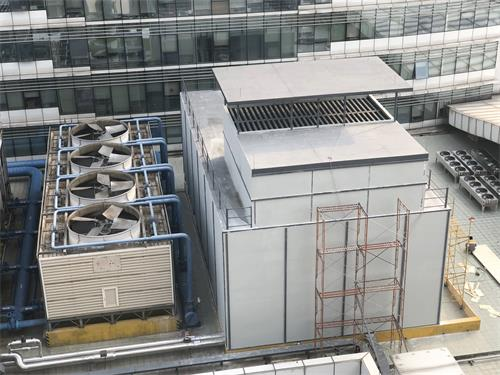1.设备类型需要冷却

2.冷却量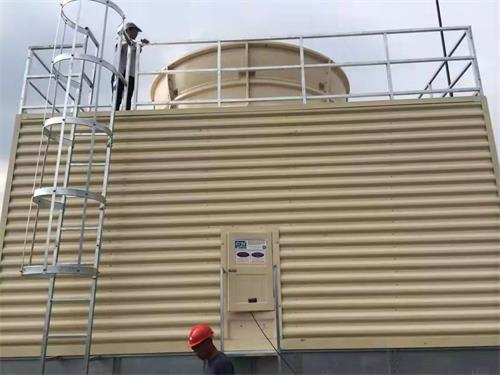1、DFNL-100:L代表无集水盘

h1=4270mm，B=2600mm，G=62000m/h，h2=1800mm，N=3KW，z=1.9T，y=3.33T，K=62kpa，Dm=59，10m=52，16m=47，De=3.02m

2、DFNDP-100:DP代表浅集水盘

h1=4890mm，B=2600mm，G=62000m/h，h2=1800mm，N=3KW，z=2.16T，y=4.24T，K=62kpa，Dm=59，10m=52，16m=47，De=3.02m

3、DFNGP-100:GP代表深集水盘

h1=5190mm，B=2600mm，G=62000m/h，h2=1800mm，N=3KW，z=2.46T，y=6.06T，K=62kpa，Dm=59，10m=52，16m=47，De=3.02m

### 相关文章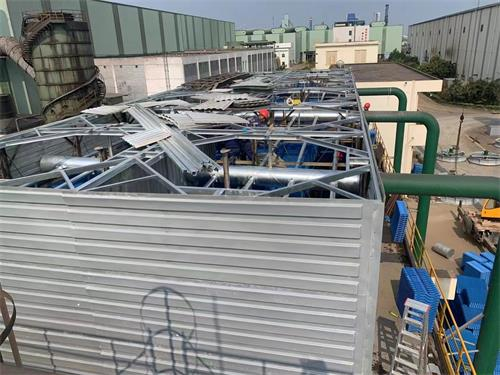##### 冷却塔是干什么用的,冷却塔的作用是什么及冷却塔的型号分类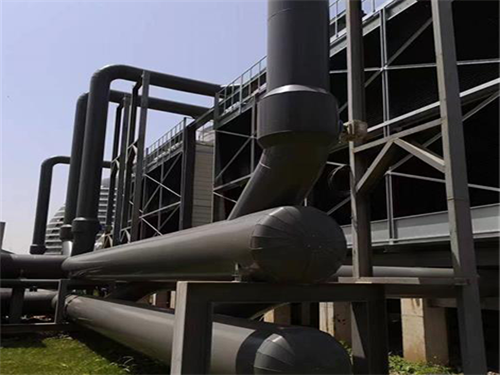##### 高效率冷却塔机房是什么,制造企业眼中的“高效机房”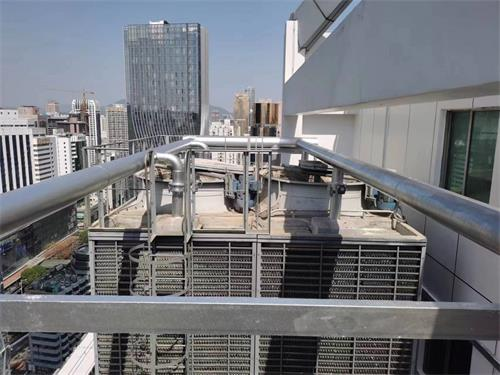##### 海外联谊大厦空调管道更换工程,中央空调管道更换和清洁的重要性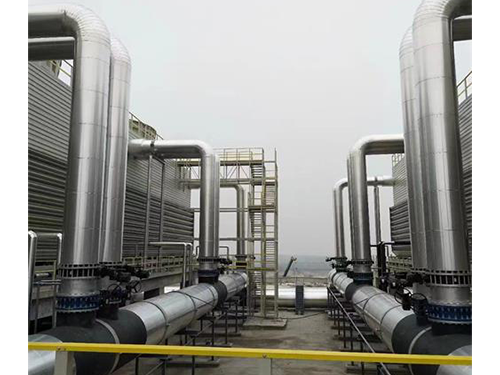##### 高效机房冷却塔有什么优势？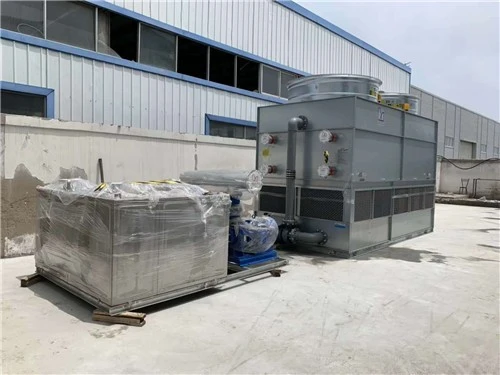##### 冷却塔漏水怎么去处理(冷却塔底部漏水如何修补)